# Introduction and Use Case:

Whether you’re new on the SOC or a seasoned Sentinel Ninja, here are some basic queries I keep coming back to when investigating anything odd about my ingest patterns (and thus my overall cost).

# Query Breakdown

So how do you know something is “odd” with your ingestion volume? I look for sudden changes. Let’s look at the ingest pattern over the past quarter and graph billable volume via the usage table with the following query for a birds-eye view of what’s going on in any environment:

``````Usage
| where TimeGenerated > ago(90d)
| where IsBillable == true
| summarize TotalVolumeGB = sum(Quantity) / 1000 by bin(StartTime, 1d), Solution
| render columnchart
``````

How does one construct such a handy query from scratch? Let’s break it apart line by line:

``````Usage                               // <--tells us which table to apply this query to. In this case it's the Usage log table.

| where TimeGenerated > ago(90d)   // <-- how far back the query will look in the table

| where IsBillable == true          // <-- filters out non-billable data (we're only worried about data that incurs a cost)

| summarize TotalVolumeGB = sum(Quantity) / 1000 by bin(StartTime, 1d), Solution    // <--Convert to GB and return results by day, per ingest solution (LogManagement, Security, etc.)

| render columnchart                // <-- graph results to a column chart
``````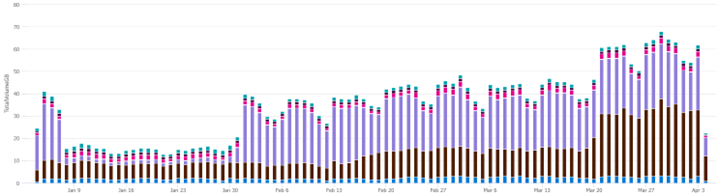# How Verbose is a Table?

``````AzureDiagnostics        // <--Define the table to query
| summarize count() by bin(TimeGenerated,1d)    // <--Return count per day
| render columnchart        // <--Graph a column chart
``````

Notes:

• By changing Line 1, you can run the query against different tables without re-writing the entire query

• Notice that compared to the previous query, the time frame isn’t specified. This was done in the GUI with a custom range as follows: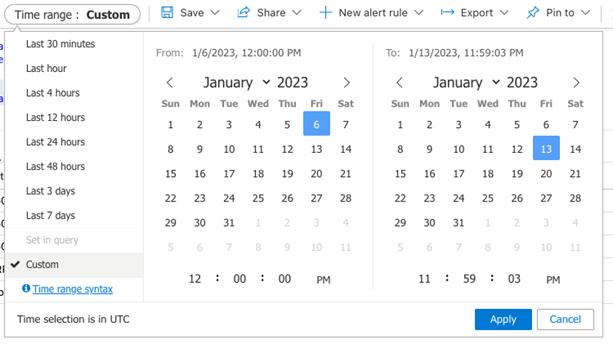Results: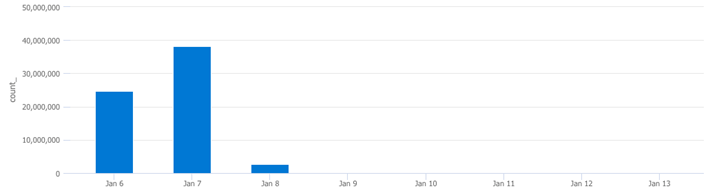# Syslog Activity by Device:

``````Syslog      // <--Define the table to query (Syslog)
| summarize count() by Computer     // <--Return Syslog count per computer
``````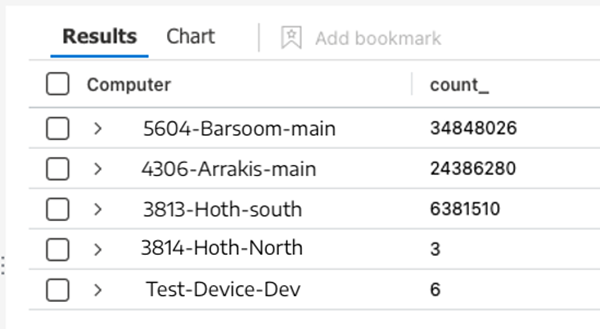# Syslog Activity per Day from a Specific Device:

``````Syslog      // <--Define the table to query (Syslog)
| where TimeGenerated > ago(90d)      // <-- how far back to query the table
| where Computer == "5604-Barsoom-main"         // <--Query a specific device
| summarize count() by bin(TimeGenerated,1d)    // <--Return count per day
| render columnchart     // <--Graph results to chart
``````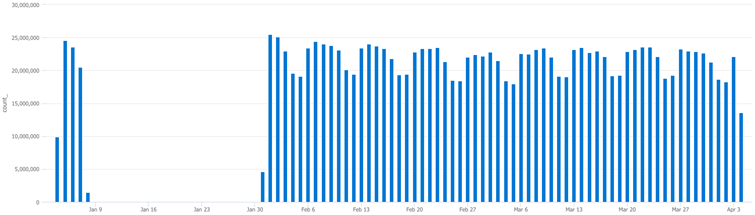# Summary:

In this post, we broke down some helpful, basic KQL queries and syntax:

• Defining table to query against
• Defining time periods manually and via GUI
• Filtering out non-billable query results
• Leveraged the Summarize function to manipulate results
• Graphing results to chart
• Querying specific devices
• Querying the Usage table for anomalies